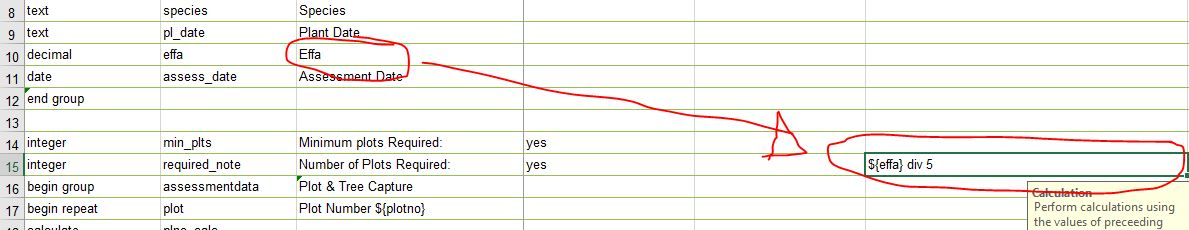# How to always round calculated integer UP to the next whole integer

604
6
11-04-2019 05:47 AMNew Contributor

Hi there

I have a decimal field that I have calculated from the input that the field worker gave in another field. It is basically a calculation where the area entered by the field worker is divided by 5. The result of this calculation needs to be rounded UP to the nearest integer. So if the answer is 5.1, then the output must round up to 6.

How can I format this output to always round up to the next integer, no matter what the decimal value says? Obviously if the decimal value is 0 then the number won't round up.

Tags (2)
6 RepliesbyMVP Notable Contributor

You could make it integer so they cannot type in a .?

Or a calculation of

if(\${field}=0,0,int(\${field})+1)byEsri Regular Contributor

add 0.5 before you cast to int.byMVP Notable Contributor

Why?  That would mess up a value of say 5.9.New Contributor

Hi Doug, thanks for the reply. With the calculation that you gave me, I am not totally sure how I should use it in my case. I already have a calculation that I am doing in that field. You will see what I mean in the attached image. Can I use both expressions together? You will see that I am already dividing the input from a previous field to give me my answer. It is this answer that I need to have rounded up all the timePS: I managed to get the formula you gave me working for my application, but the problem is that it add 1 to the answer even if it is a whole number. This means that if someone inputs 25 (which should give a result of 5), now gives you a result of 6 because 1 was added on to 25 to give you 26. This divided by 5 gives you 5.2, which then rounds up to 6 using the formula.

if((\${effa} div 5)=0,0,int((\${effa} div 5))+1)

I have also been playing with a mod and round expression, and I seem to have gotten it to work for me:

if((\${effa} div 5)  mod 1 < 0.5, round((\${effa} div 5) + 0.5, 0), round((\${effa} div 5), 0))byEsri Notable Contributor

Hi.  This seems to be working for me. It is basically the same thing Doug posted plus a little twist to handle situations where people enter an integer value.

typenamelabelcalculationbind::type
decimaleffaInput
calculateeffa5\${effa} div 5decimal
integeroutputOutputif(int(\${effa5})=\${effa5},\${effa5},int(\${effa5})+1)

The XLSForm above will add a field into your feature layer called effa5. If you do not want that, simply set the bind::esri:fieldType column to null for effa5.New Contributor III

@Paulvan_Heerden1 it would be nice if survey123 had a RoundUp function. I have posted it as an idea , please kudo it up if you think it would be helpful